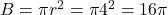## The radius of a cylindrical water tank is 4 ft, and its height is 6 ft, what is the volume of the tank?

Question

The radius of a cylindrical water tank is 4 ft, and its height is 6 ft, what is the volume of the tank?

in progress 0
2 months 2021-07-24T15:23:58+00:00 2 Answers 0 views 0

96π cu ft.

Step-by-step explanation:

V = Bh      B = area of the base     h = height

The base is a circle, soV = 16π(6) = 96π cu ft.